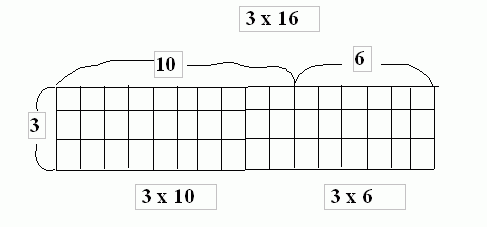Email us to get an instant 20% discount on highly effective K-12 Math & English kwizNET Programs!

#### Online Quiz (WorksheetABCD)

Questions Per Quiz = 2 4 6 8 10

### Grade 3 - Mathematics9.5 Multiplying Two Digit with One Digit Number (WIZ Math)

3 x 16 = ?
One way to multiply 3 by 16 is to break into smaller numbers. So it can be written as:
3 x 16 = (3 x 10) + (3 x 6) = 30 + 18 = 48Short Method:

1. Multiply the single digit by both the digits of the 2 digit number.
2. If the product in tens place is a 2 digit number, we carry the tens digit of the number to the tens place of the factors.

Example:
 Question Step 1 Step 2Method:

Example:
 Question Step 1 Step 2 Step 3Directions: Find the product of the following. Also write at least 10 examples of your own.
 Question 1: 93 * 8

 Question 2: 76 * 9

 Question 3: 72 * 7

 Question 4: 80 * 4

 Question 5: 96 * 9

Question 6: This question is available to subscribers only!

Question 7: This question is available to subscribers only!

Question 8: This question is available to subscribers only!

Question 9: This question is available to subscribers only!

Question 10: This question is available to subscribers only!